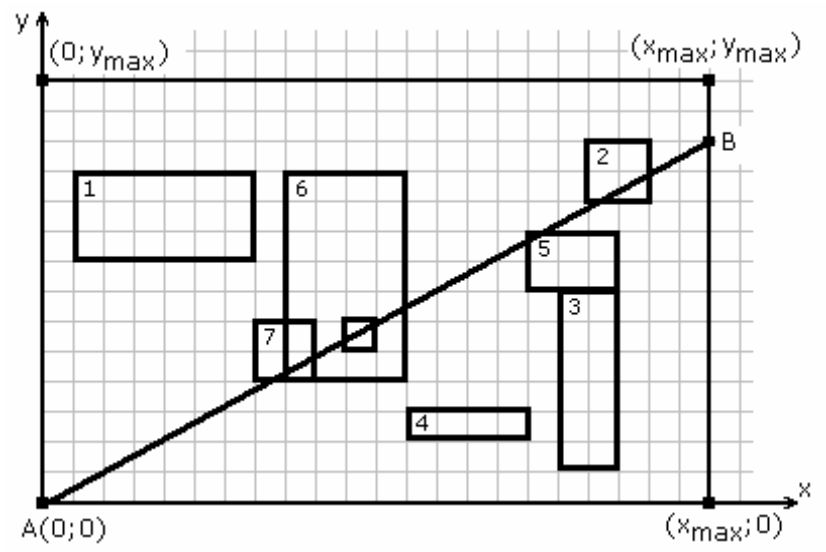시간 제한메모리 제한제출정답맞힌 사람정답 비율
1 초 512 MB49000.000%

## 문제

There are given N rectangles on the plane. Rectangle sides are parallel to coordinate axis. These rectangles may overlap, coincide or be drawn inside one another. Their vertices have non-negative integer coordinates and x coordinates do not exceed xmax and y coordinates do not exceed ymax.

A segment is started in the point A(0, 0) and ended in point B. The coordinates of the point B (the other end of the segment) satisfy the following conditions:

• The coordinates of B are integer numbers;
• The point B belongs either to the segment [(0, ymax), (xmax, ymax)] or to the segment [(xmax, 0), (xmax, ymax)];

The segment AB might cross rectangles (we assume that crossing takes place even if only one rectangle vertex is crossed).

Write a program to find a point B for which the segment AB crosses as many rectangles as possible.Example with 8 rectangles. The segment AB crosses 5 of them.

## 입력

The first line of the input contains three integers: xmax, ymax (0 < xmax, ymax ≤ 109) and N (1 ≤ N ≤ 10000). Each of the following N lines contains four integers: coordinates of the bottom left corner xbl and ybl and coordinates of the top right corner xtr and ytr. Neighbouring numbers are separated by single space character.

## 출력

On the first and only line of the output three integer numbers should be written. First – the maximum number of crossed rectangles followed x and y coordinates of point B. Neighbouring numbers must be separated by single space character.

If there are several solutions, find any one of them.

## 예제 입력 1

22 14 8
1 8 7 11
18 10 20 12
17 1 19 7
12 2 16 3
16 7 19 9
8 4 12 11
7 4 9 6
10 5 11 6


## 예제 출력 1

5 22 12


## 힌트

Another possible solution is 5 22 11